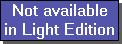The SDL Component Suite is an industry leading collection of components supporting scientific and engineering computing. Please visit the SDL Web site for more information....## VarYUnit: SDL_statis Class: TPLSModel Declaration: property VarY: TVector;

The readonly vector property VarY returns the amount of explained variance of the Y matrix (the explained Y variance specifies the amount of variation in the response variables which is covered by the model).

The vector contains the explained variances in order of the factors, i.e. the first vector element holds the explained variance of the first factor, the second vector element the explained variance of the second factor, and so on.

The explained variances are scaled to a sum of 1.00. Thus the percentage of explained variance can be obtained by multiplying the vector elements by 100.

Last Update: 2012-Oct-20